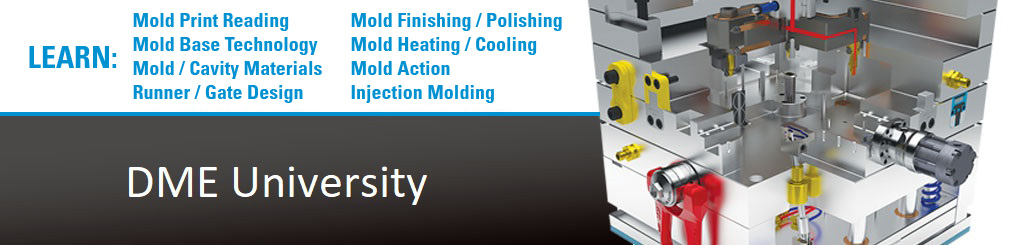# Convert A Fraction To A Decimal

Pick a Fraction from the ruler. Using your calculator, divide the Numerator (the top number) by the Denominator (bottom number).

The result is a Decimal number. It should match the appropriate number in the Decimal column.

Previous

Next

Page 2 of 32